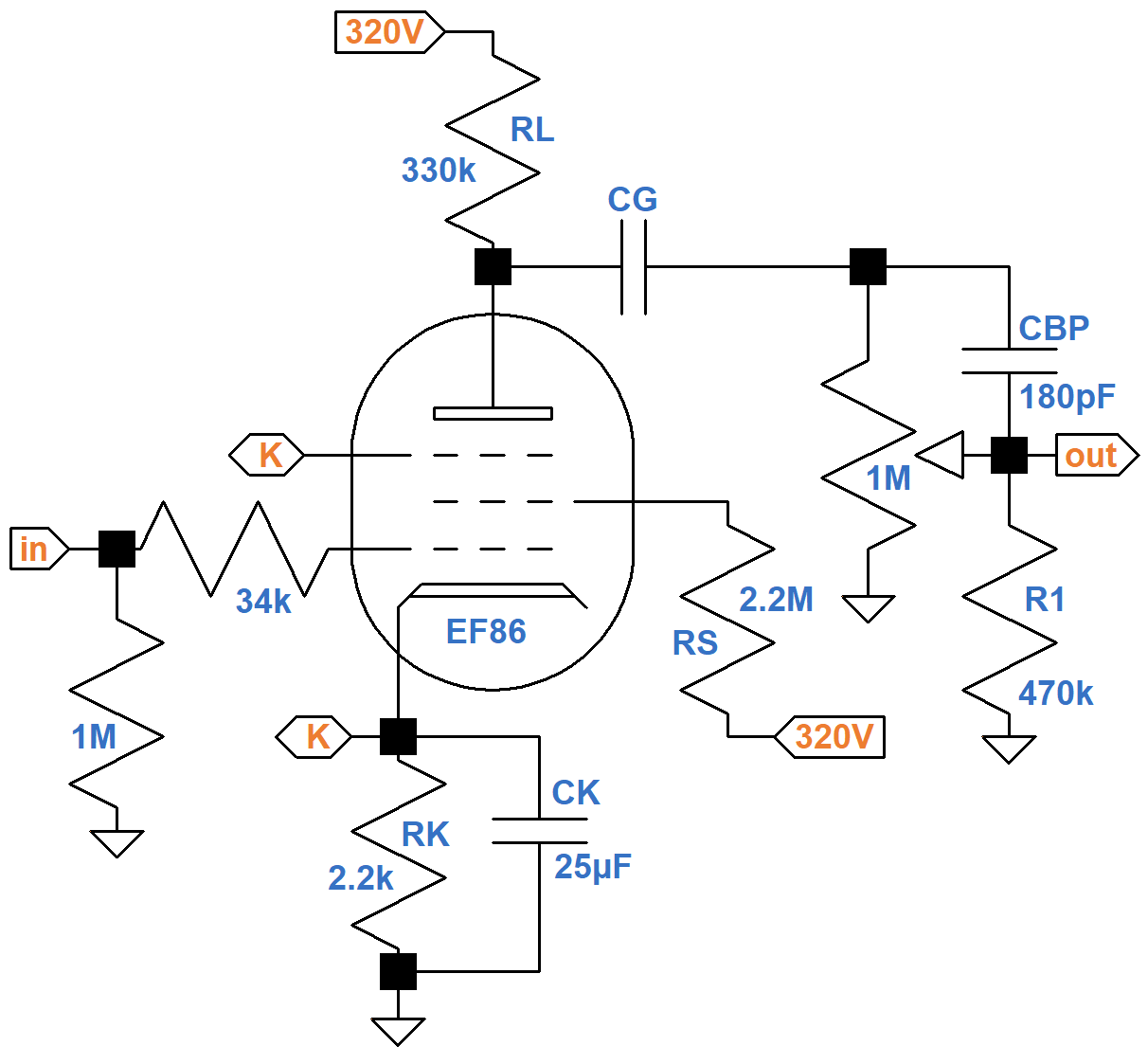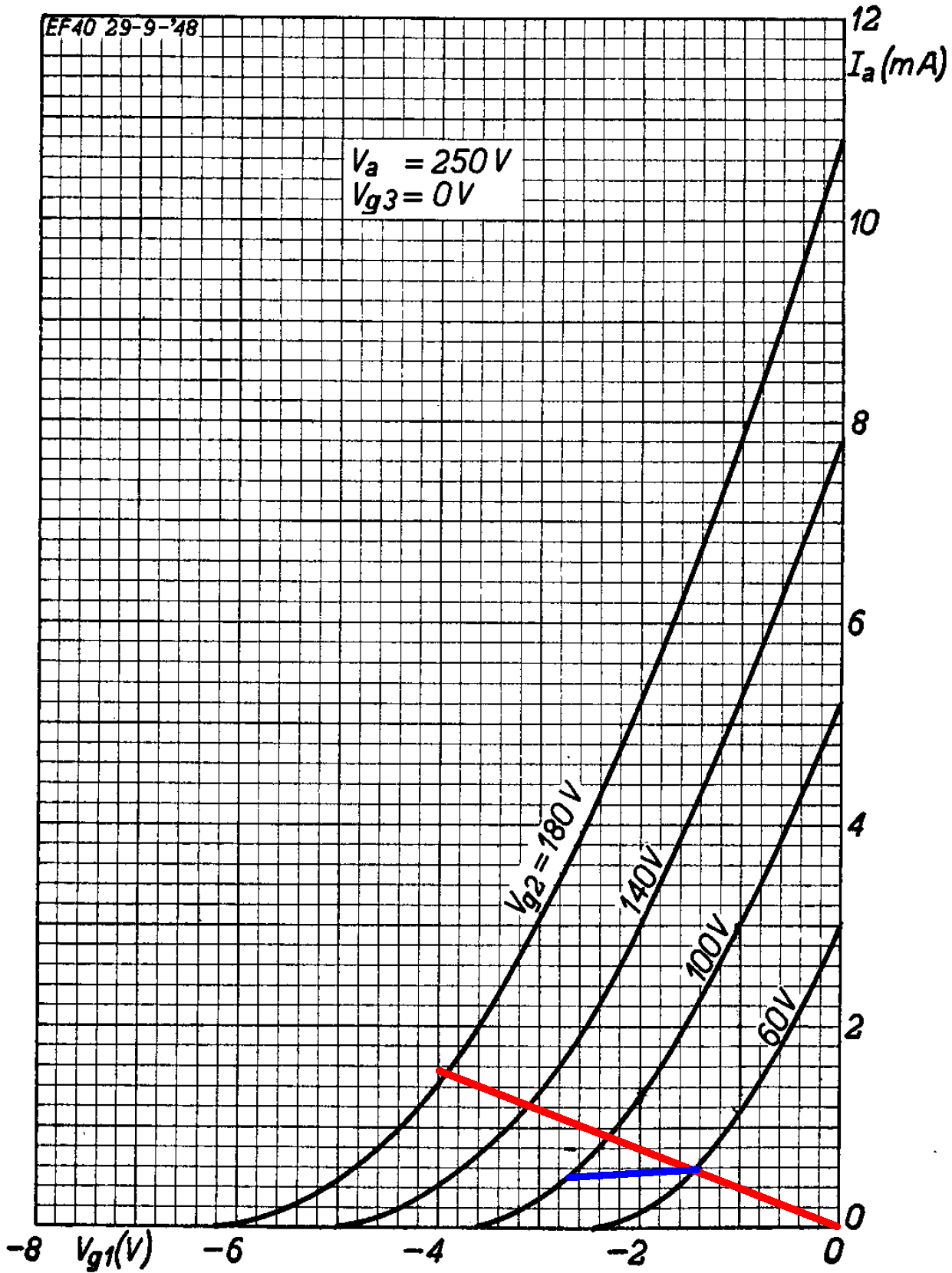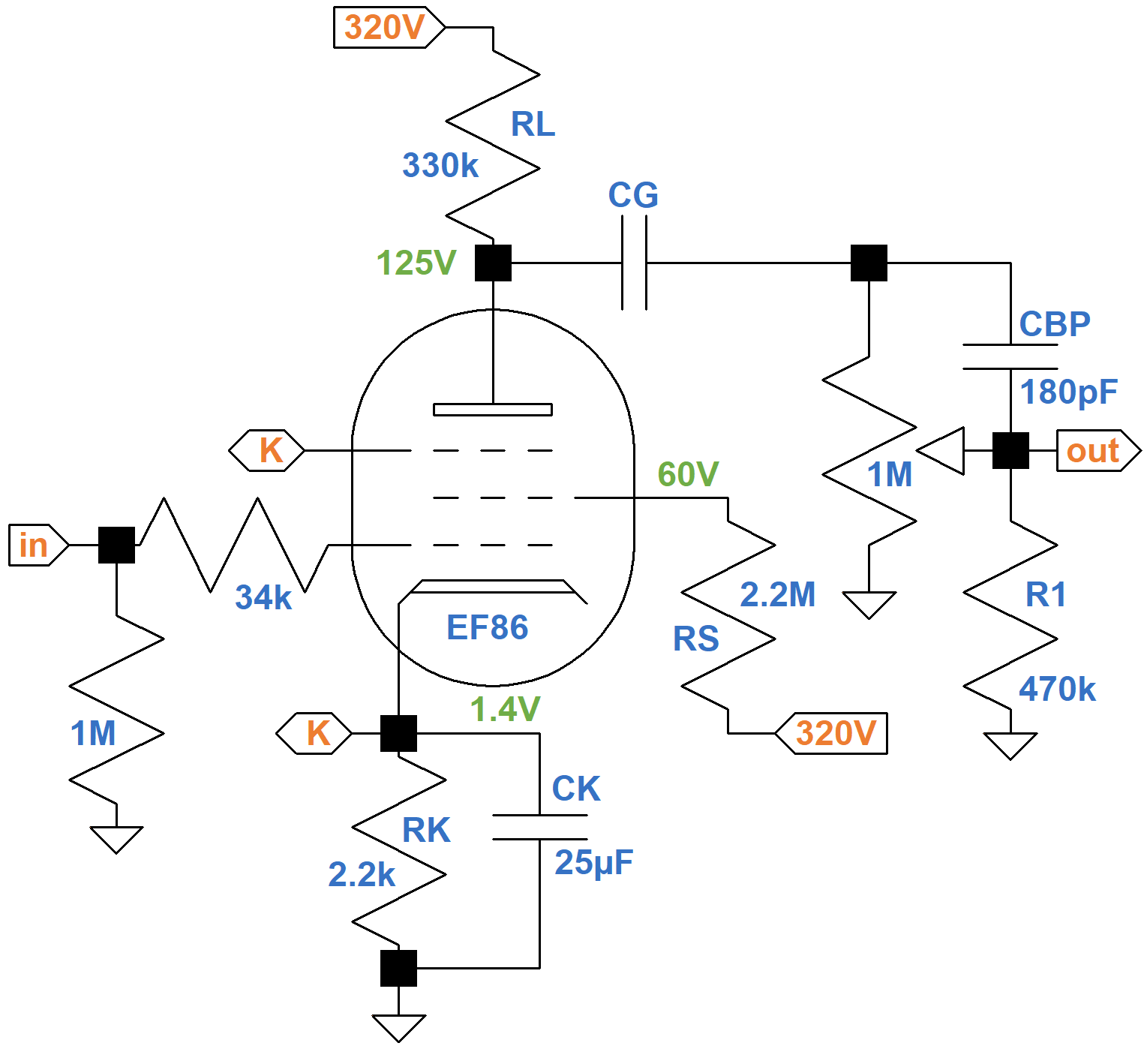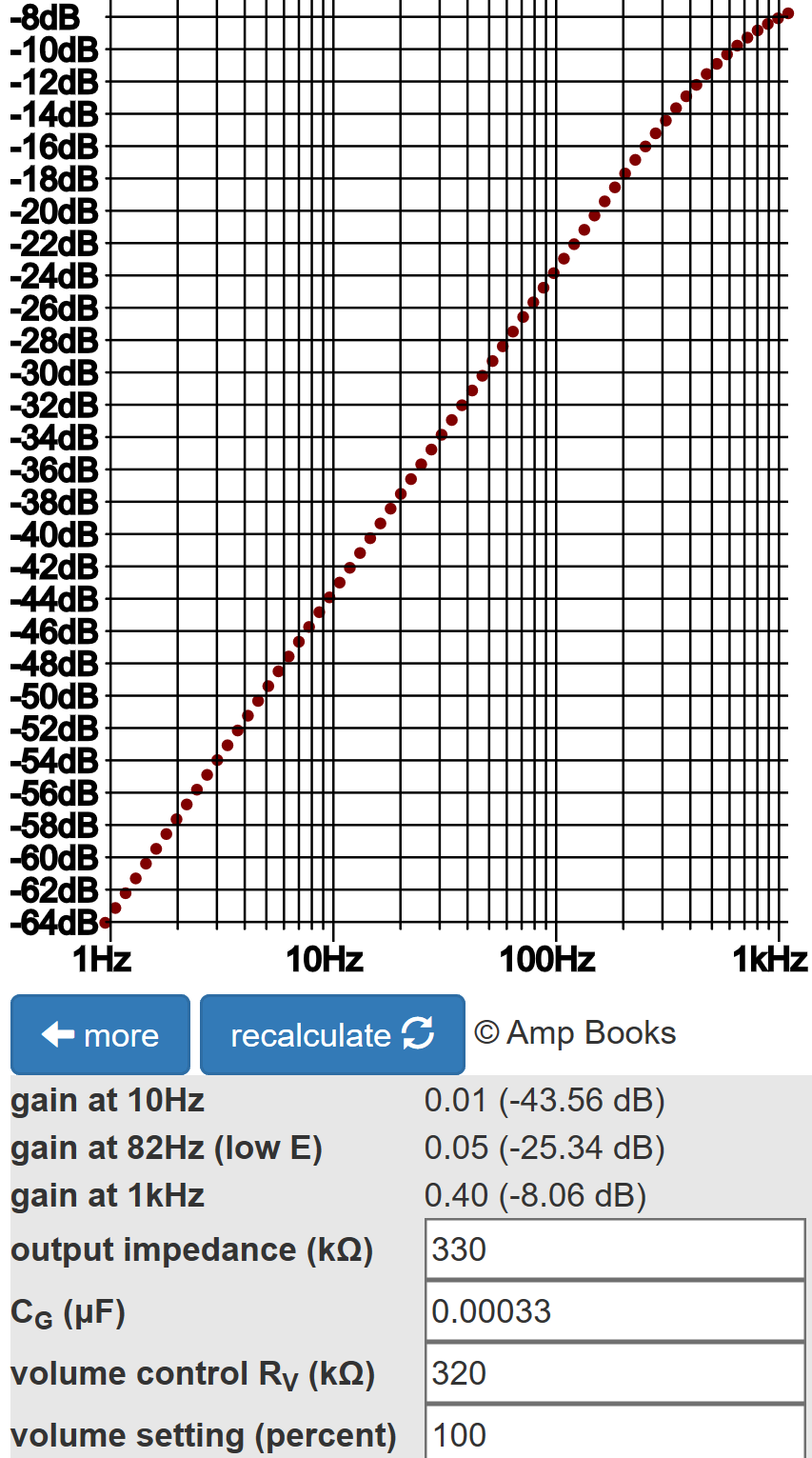"As used in the DC-30 - and the Vox AC15 (and briefly, the early AC30) that inspired it - an EF86 pentode preamp tube helps to create a sound in the amp's second channel that is really quite different from the classic Top Boost chime and shimmer most consider the 'classic Vox sound.' This tube yields a thick, rich tone with a full and relatively even reproduction of the frequency spectrum (as relates to the electric guitar)..." 1

The first stage for Channel 2 of the Matchless DC-30 guitar amplifier contains an EF86 pentode that drives the effects send or the phase inverter via a switchable coupling capacitor CG and a 1MΩ volume control.CG is switched in 6 steps between 330pF (maximum bass cut) and 0.01μF (minimum bass cut).

The AC load varies from 1MΩ||470kΩ = 320kΩ (volume at maximum) and 1MΩ (volume near minimum, low frequencies, for which CBP acts as an open circuit). Because the plate resistance of a pentode is very high, the output impedance of a pentode voltage amplifier is approximately equal to the plate load resistor value RL. By setting RL equal to 330kΩ, Matchless creates an output impedance that approximately matches the lower limit of the AC load.Master the basics of preamp, power amp, and power supply design

#### DC Operating Point

According to Ohm's Law, if the voltage across the 2.2kΩ cathode resistor is 4V, then the cathode current is

4V / 2.2kΩ = 1.82mA

The represents plate current plus screen current. According to the data sheet, the ratio of plate current to screen current is typically 5, so the plate current is 5/6 of this value: 1.52mA.

If the voltage across the cathode resistor is zero, the plate current is zero. The endpoints for the red line shown here on the EF86 plate transfer characteristics are -4V, 1.52mA and 0V, 0mA.If the screen voltage is 100V, the voltage drop across the 2.2MΩ screen resistor is 320V - 100V = 220V. According to Ohm's Law, the screen current through the resistor is 0.1mA, so the plate current is 5 times greater: 0.5mA. Repeating this logic for a 60V screen, the plate current is 0.59mA. These are the endpoints of the blue line. The lines intersect at a 60V screen.Design your amp using a structured, professional methodology

According to the transfer characteristics, the DC grid bias is -1.4V. The DC plate current is 0.59mA, so the voltage drop across the 330kΩ plate load resistor is

(0.59mA)(330kΩ) = 195V

The plate voltage is therefore 320V - 195V = 125V. Here are our estimates of the DC voltages based on an average tube.Know it works by measuring performance at every point in the amplifier

#### Voltage Gain

Based on the data sheet's typical transconductance of gm = 2mS ("S = 2ma/V"), unloaded voltage gain is gmRL = (2mS)(330kΩ) = 660 (56dB), which is impressive - try getting that from a triode! The output impedance is approximately 330kΩ, however, so when the AC load is 320kΩ, voltage gain is reduced by a factor of 320kΩ / (320kΩ + 330kΩ) = 0.49 (-6.2dB). Gain is still much greater than for a typical 12AX7 stage.

#### Switched Tone Control

Depending on switch position, CG takes on these values: 330pF, 560pF, 0.0012μF, 0.0022μF, 0.0047μF, and 0.01μF. According to the Coupling Capacitor calculator, bass attenuation is most severe with the volume control at maximum (320kΩ AC load) and CG = 330pF. In fact, there is 8dB attenuation at 1kHz.With the knob fully clockwise, the coupling capacitor value is 0.01μF and there is only 0.4dB attenuation at 82Hz compared to 1kHz.#### Reference

1Dave Hunter, Amped, (London: Voyageur Press, 2012), p. 199.Master the basics of preamp, power amp, and power supply designDesign your amp using a structured, professional methodologyKnow it works by measuring performance at every point in the amplifierThe Fender Bassman 5F6-A (plus Marshall's Bluesbreaker and Plexi)Georg Simon Ohm - imagine a world without Ohm's Law!